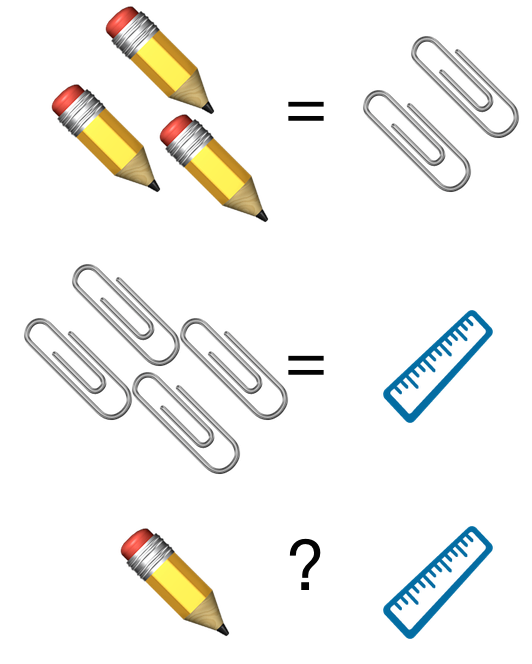# Let's Barter!

Algebra Level 1Three pencils cost the same as two paper clips.
Four paper clips cost the same as one ruler.
Pencils are more expensive than rulers.

If the first two statements are true, the third statement is $\text{\_\_\_\_\_\_\_\_\_}$.

Note: Assume that each item costs some positive amount.

×